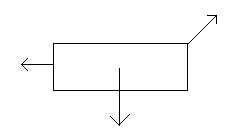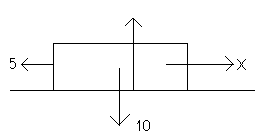## Newton's Laws of Motion

Newton's laws of Motion covers Newtons three laws.

Forces

A force is "an influence tending to cause the motion of a body" (Oxford English Dictionary).

Forces are usually represented diagrammatically as an arrow, pointing in the direction the force. For example:Force is measured in newtons (symbol N).

Newton's First Law

• Newton's First Law of Motion states that a body will remain at rest or will continue to move at a constant velocity, unless an external force is applied.

This means that in order for the acceleration of a body to change, there must be a net force applied to the body. Put another way, if the forces on an object balance, there will be no acceleration (the object will continue at the same speed).

So, if we are told that a body is not accelerating (i.e. if it is moving at a constant velocity), we know that the resultant (overall) force in any one direction will be zero.

Example

The following forces are acting on a body. The body moves at a constant speed of 5m/s. Find force X.It should be clear that X = 5. The force is therefore 5N.

Newton's Second Law

• Newton's Second Law of Motion states that the rate of change in momentum of the body is directly proportional to the net force applied.

In other words, when an overall force is applied to an object, the acceleration will change. By how much the acceleration changes depends upon the magnitude of the force applied.

In fact, from Newton's Second Law we can derive the following equation:

• Resultant Force on Body = Mass of Body × Acceleration of Body

This is sometimes written as F = ma, though you should make sure you understand what this means (in particular, note that F is resultant force).

Weight and Mass

Students are often confused about the difference between weight and mass. Weight is the force due to gravity and is measured in newtons. Mass is the amount of matter that a body contains and is measured in kilograms (kg). Weight and mass are related by the equation:

• W = mg

This is a consequence of Newton's Second Law.

Newton's Third Law

• This law states that every action has an equal and opposite reaction.

For example, if a ball is placed on the table, the ball will exert a force on the table. At the same time, however, the table exerts a force on the ball (it is this force that prevents the ball from being sucked into the table!).

This "equal and opposite reaction force" is known as the normal reaction force, and the letter N or R is commonly used to represent it.